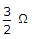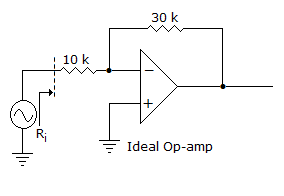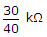# Electronics and Communication Engineering - Analog Electronics

26.

Two 3Ω speakers in series will have a total impedance of

 A. 1 Ω B.C. 3 Ω D. 6 Ω

Explanation:

No answer description available for this question. Let us discuss.

27.

The input resistance Ri of the amplifier shown in the figureA.B. 100 kΩ C. 40 kΩ D. ∞

Explanation:

No answer description available for this question. Let us discuss.

28.

In a CE amplifier the ac signal is increased. Which of the following will not change?

 A. Ac base voltage B. Ac collector voltage C. Ac emitter voltage D. Ac collector voltage and ac emitter voltage

Explanation:

No answer description available for this question. Let us discuss.

29.

The ideal op-amp has the following characteristics

 A. Ri = ∞, A = ∞, R0 = 0 B. Ri = 0, A = ∞, R0 = 0 C. Ri = ∞, A = 0, R0 = 0 D. Ri = 0, A = ∞, R0 = ∞

Explanation:

No answer description available for this question. Let us discuss.

30.

The input differential stage of op-amp. 741 is baised at about 10 μA current such a low current of the input stage gives

1. high CMRR
2. high differential gain
3. low differential
4. high input impedance
Which of these are correct?

 A. 1 and 2 B. 1, 2 and 4 C. 3 and 4 D. 1, 2, 3 and 4